# RS Aggarwal Solutions for Class 8 Maths Chapter 17 - Construction of Quadrilaterals

RS Aggarwal Solutions for Class 8 Maths Chapter 17- Construction of Quadrilaterals, are provided here. Our expert faculty team has prepared solutions in order to help you with your exam preparation to acquire good marks in Maths. RS Aggarwal Solutions for Class 8 Maths comes in very handy at this point. Our solution module utilizes various shortcut tips and practical examples to explain all the exercise questions in a simple and easily understandable language. Practicing the textbook questions will help you in analyzing your level of preparation and knowledge of the concept.

In order to help you understand and solve the problems, we at BYJU’S have designed these solutions in a very lucid and clear manner that helps students solve problems in the most efficient ways possible. Here is the RS Aggarwal Solutions for Class 8 Chapter 17 where solutions are solved in detail. Download pdf of Class 8 Chapter 17 in their respective links.

## Download PDF of RS Aggarwal Solutions for Class 8 Maths Chapter 17 – Construction of Quadrilaterals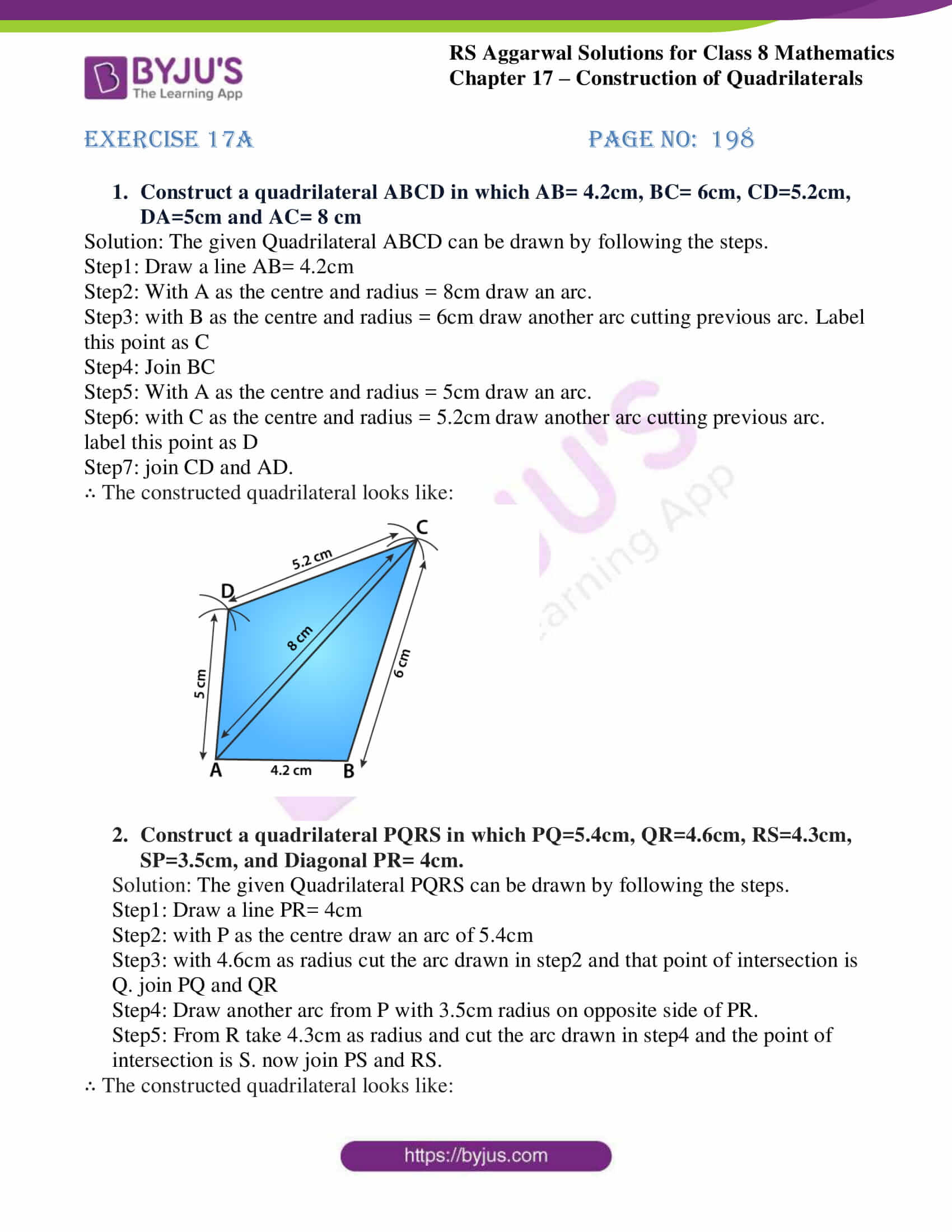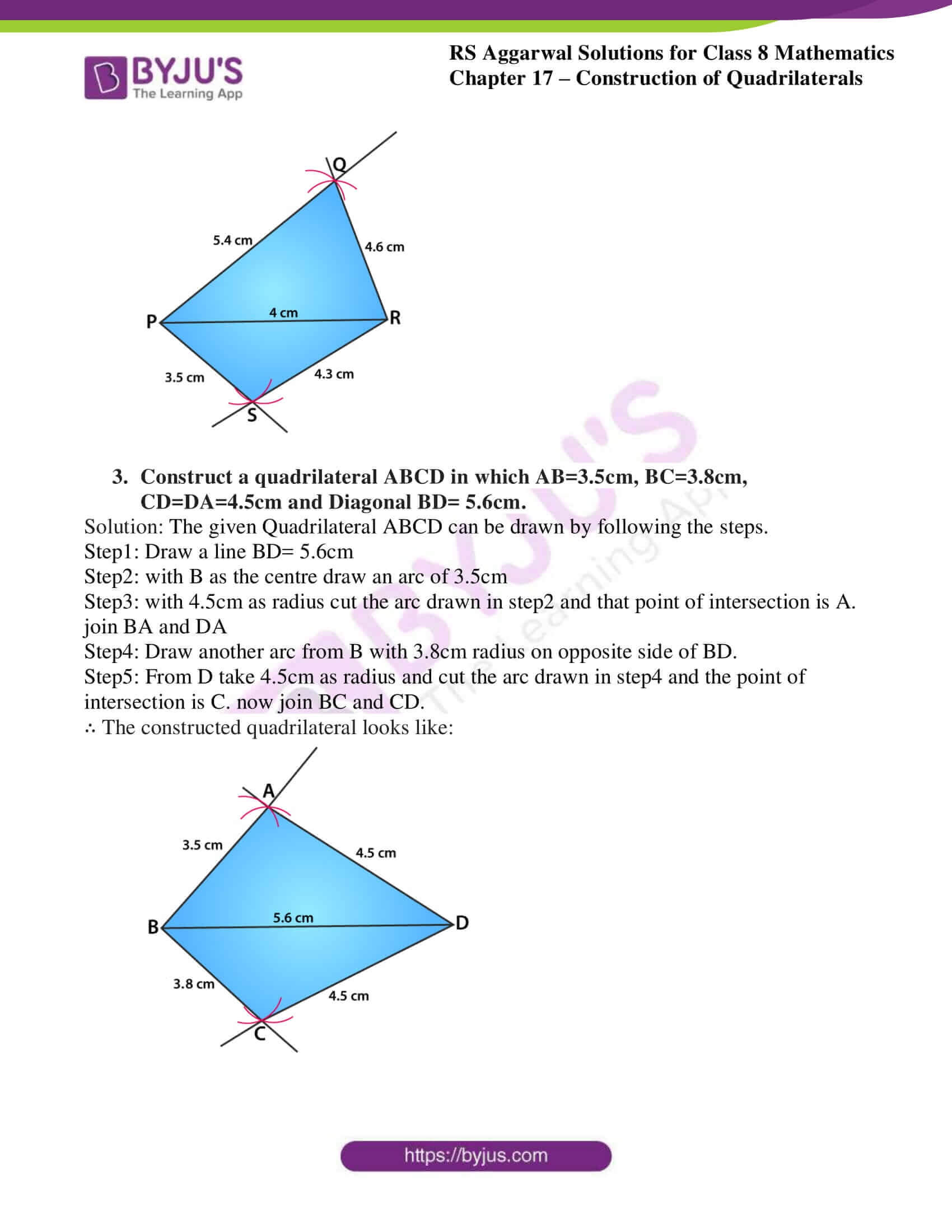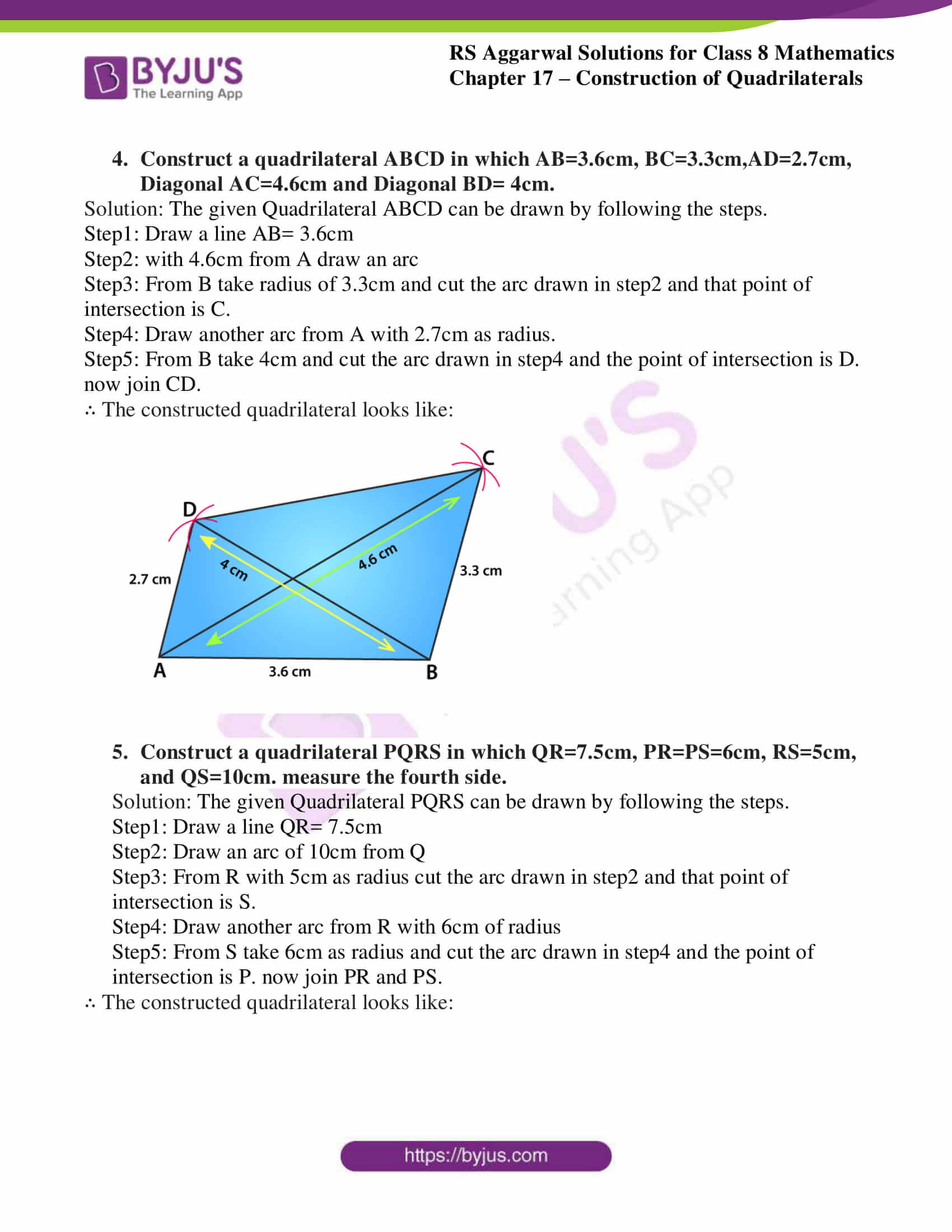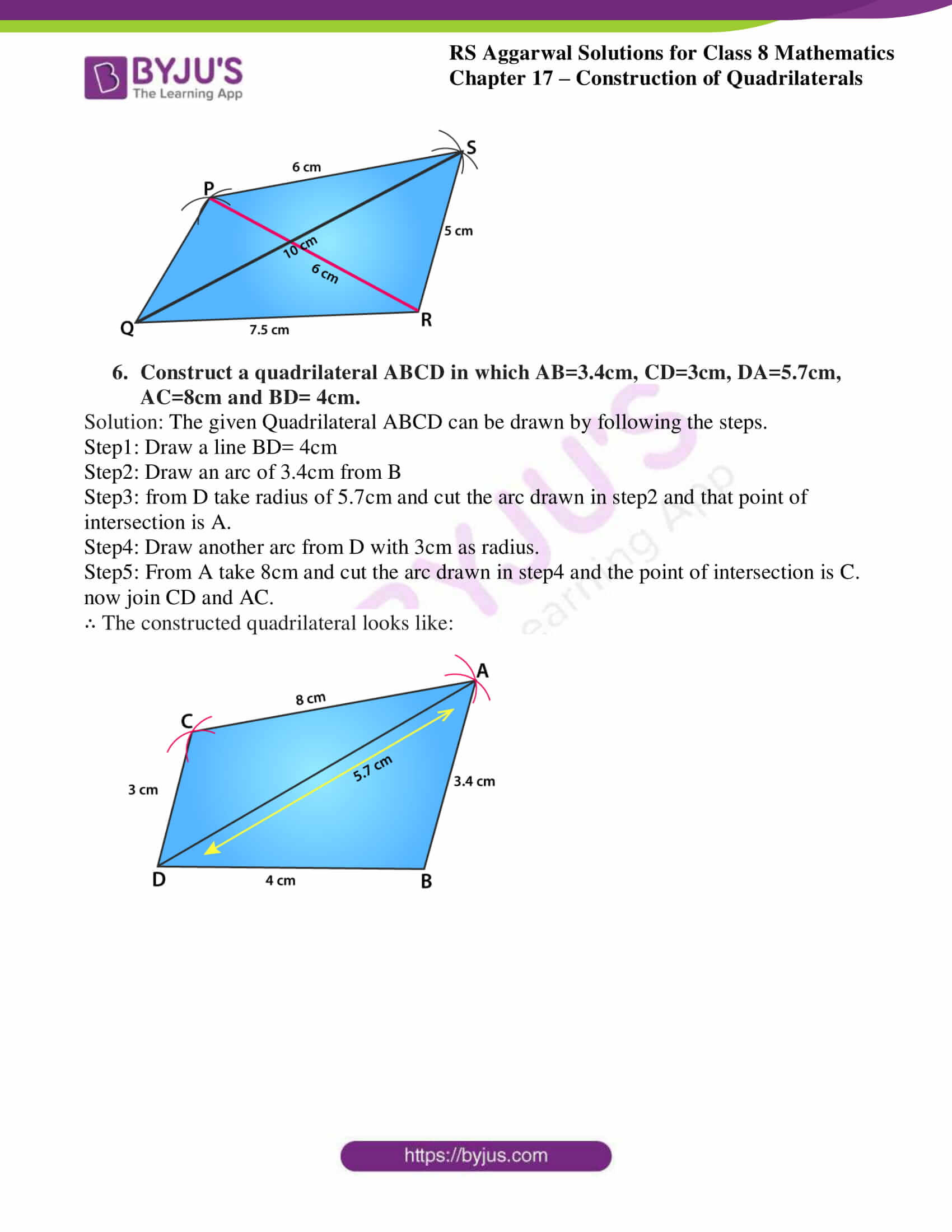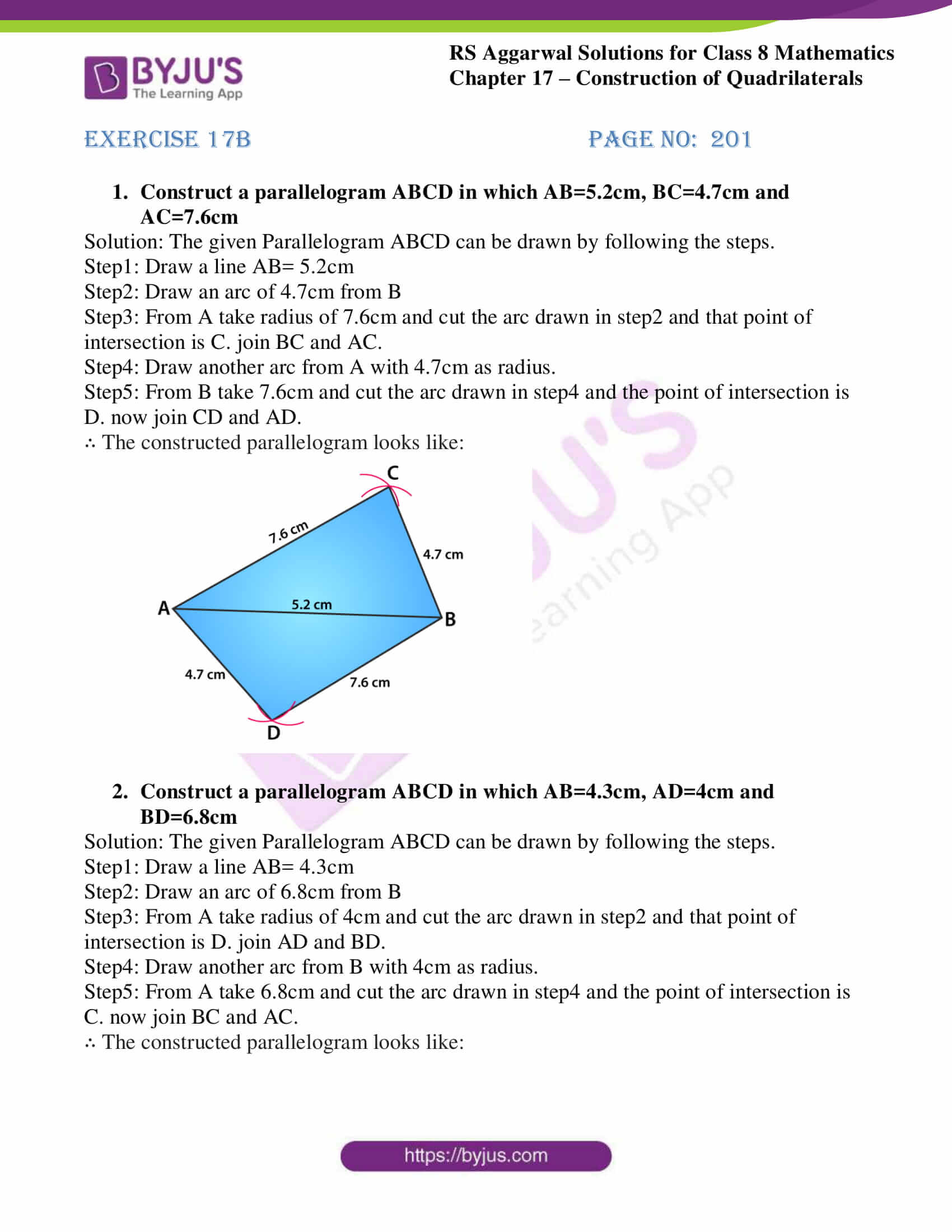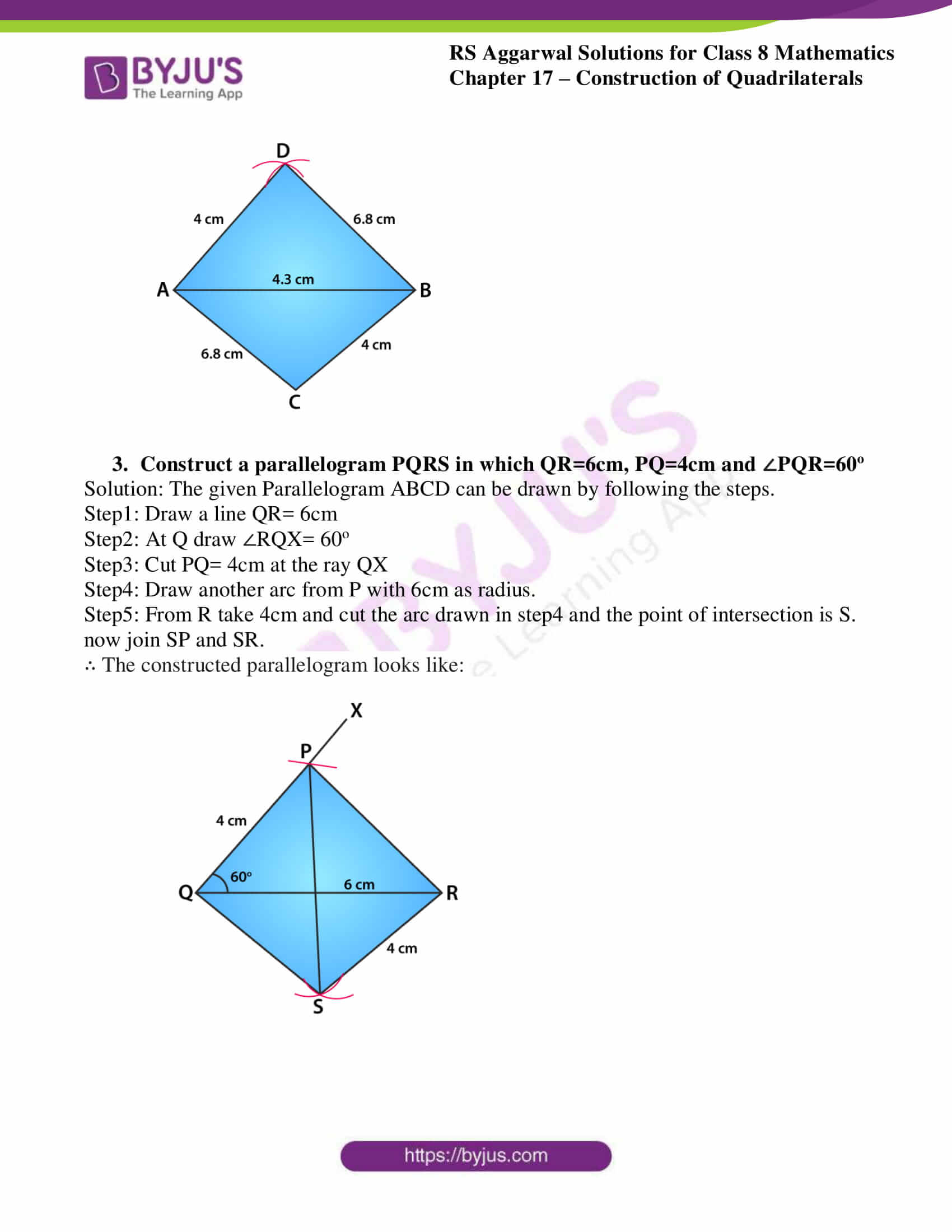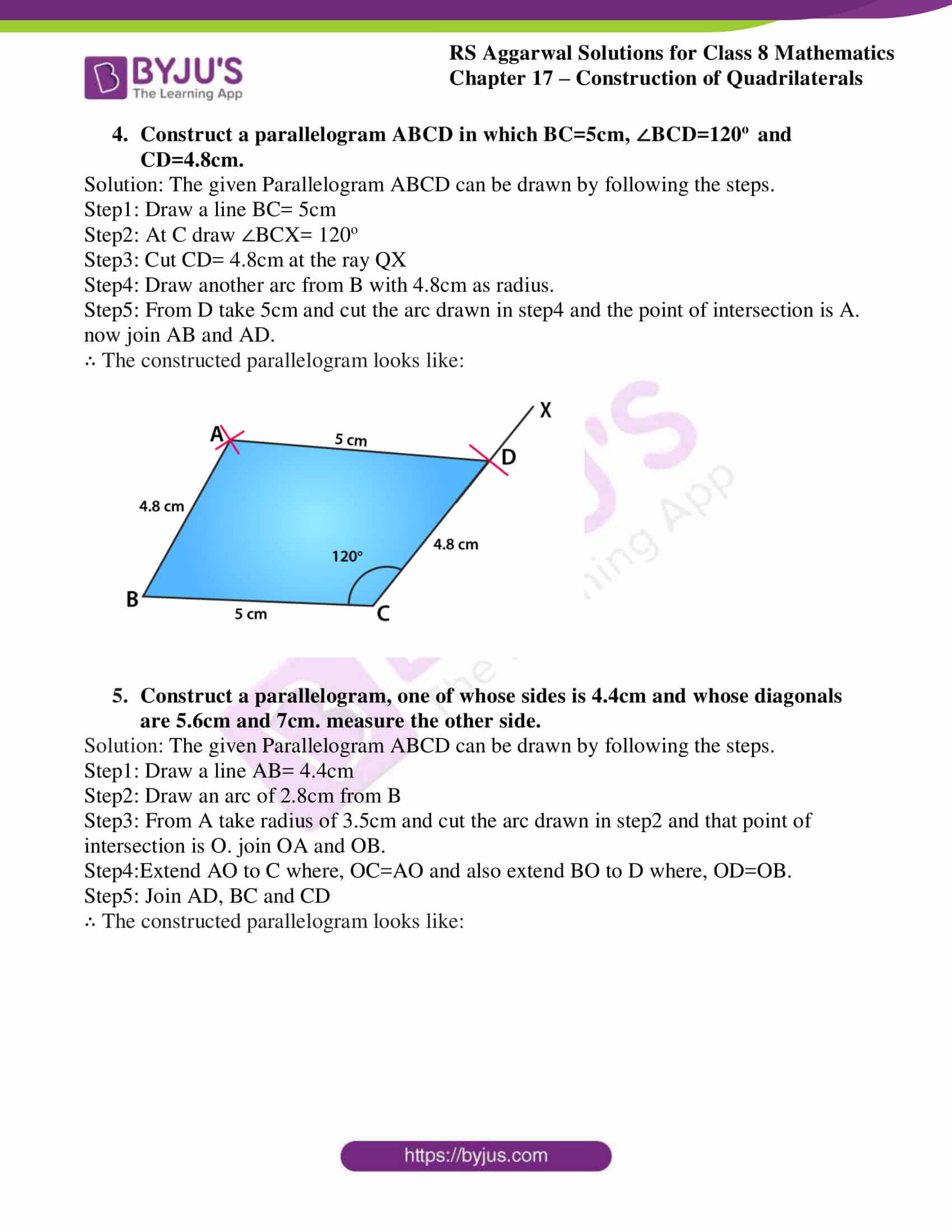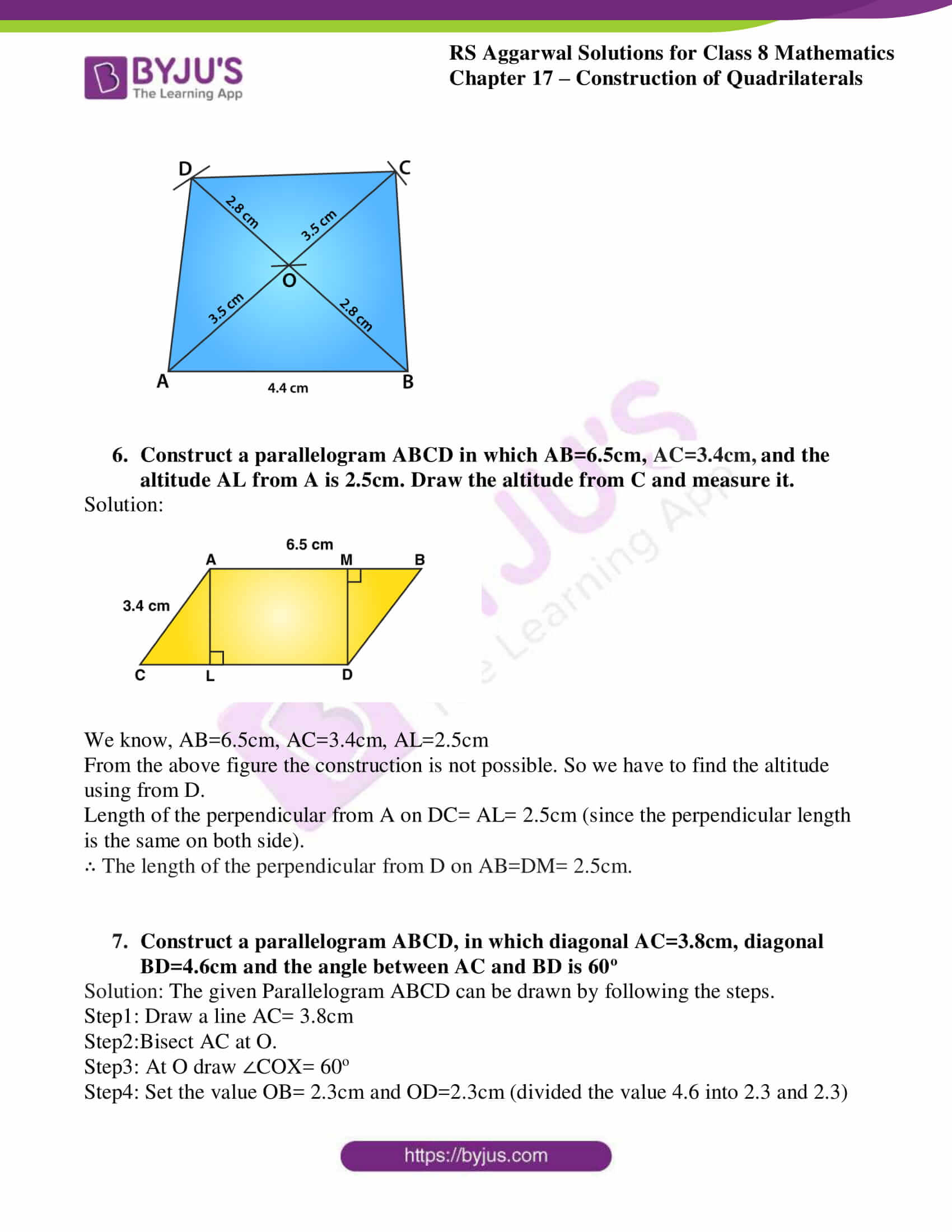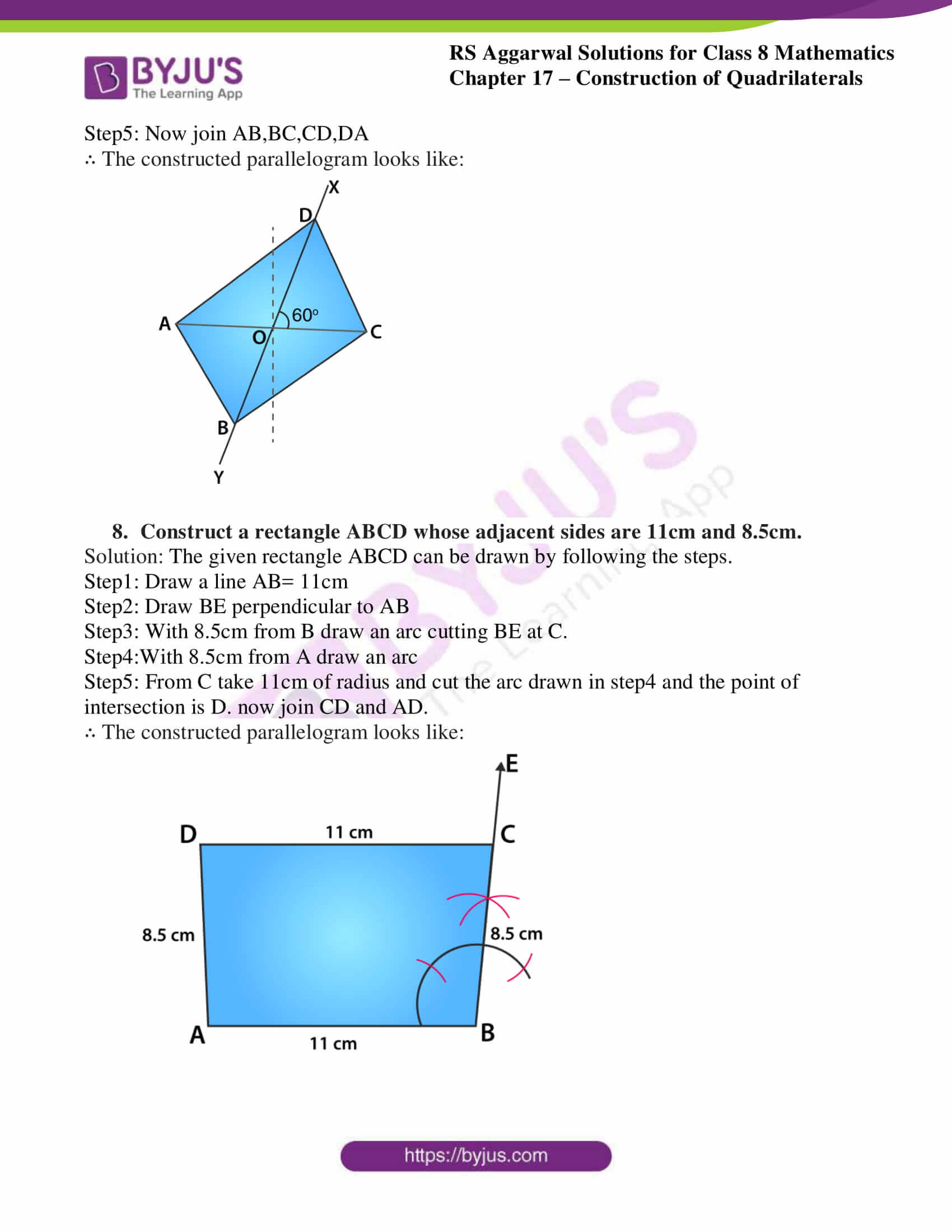## Exercise 17A

1. Construct a quadrilateral ABCD in which AB= 4.2cm, BC= 6cm, CD=5.2cm, DA=5cm and AC= 8 cm

Solution: The given Quadrilateral ABCD can be drawn by following the steps.

Step1: Draw a line AB= 4.2cm

Step2: With A as the centre and radius = 8cm draw an arc.

Step3: with B as the centre and radius = 6cm draw another arc cutting previous arc. Label this point as C

Step4: Join BC

Step5: With A as the centre and radius = 5cm draw an arc.

Step6: with C as the centre and radius = 5.2cm draw another arc cutting previous arc. label this point as D

∴ The constructed quadrilateral looks like: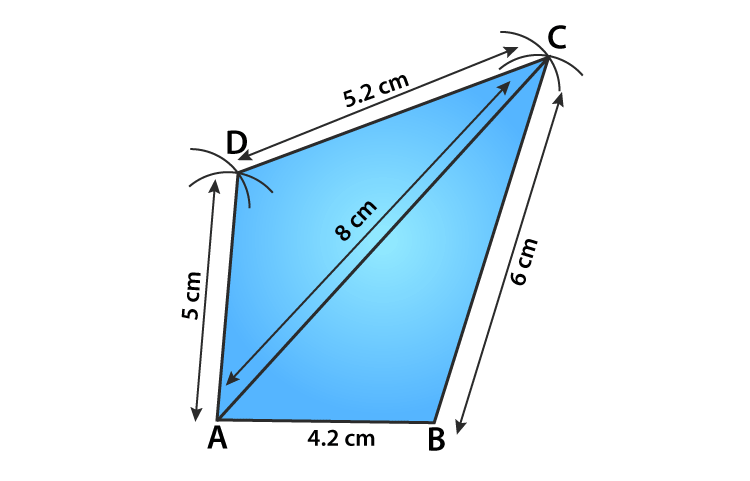2. Construct a quadrilateral PQRS in which PQ=5.4cm, QR=4.6cm, RS=4.3cm, SP=3.5cm, and Diagonal PR= 4cm.

Solution: The given Quadrilateral PQRS can be drawn by following the steps.

Step1: Draw a line PR= 4cm

Step2: with P as the centre draw an arc of 5.4cm

Step3: with 4.6cm as radius cut the arc drawn in step2 and that point of intersection is Q. join PQ and QR

Step4: Draw another arc from P with 3.5cm radius on opposite side of PR.

Step5: From R take 4.3cm as radius and cut the arc drawn in step4 and the point of intersection is S. now join PS and RS.

∴ The constructed quadrilateral looks like: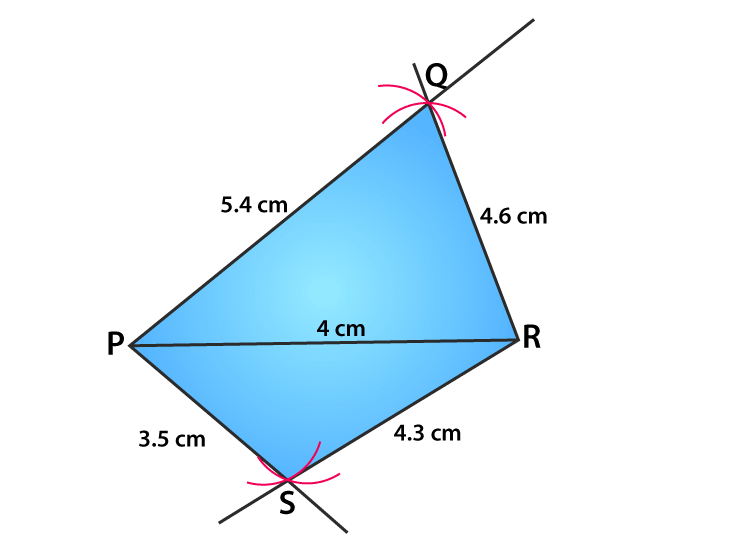3. Construct a quadrilateral ABCD in which AB=3.5cm, BC=3.8cm, CD=DA=4.5cm and Diagonal BD= 5.6cm.

Solution: The given Quadrilateral ABCD can be drawn by following the steps.

Step1: Draw a line BD= 5.6cm

Step2: with B as the centre draw an arc of 3.5cm

Step3: with 4.5cm as radius cut the arc drawn in step2 and that point of intersection is A. join BA and DA

Step4: Draw another arc from B with 3.8cm radius on opposite side of BD.

Step5: From D take 4.5cm as radius and cut the arc drawn in step4 and the point of intersection is C. now join BC and CD.

∴ The constructed quadrilateral looks like: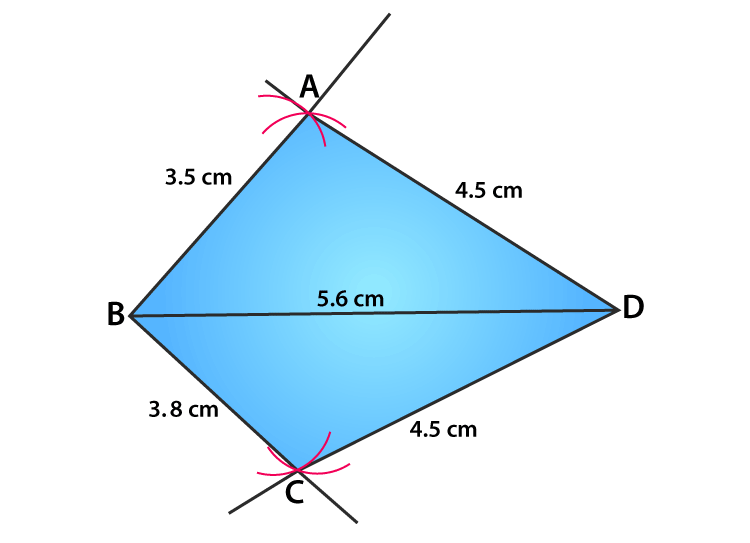4. Construct a quadrilateral ABCD in which AB=3.6cm, BC=3.3cm,AD=2.7cm, Diagonal AC=4.6cm and Diagonal BD= 4cm.

Solution: The given Quadrilateral ABCD can be drawn by following the steps.

Step1: Draw a line AB= 3.6cm

Step2: with 4.6cm from A draw an arc

Step3: From B take radius of 3.3cm and cut the arc drawn in step2 and that point of intersection is C.

Step4: Draw another arc from A with 2.7cm as radius.

Step5: From B take 4cm and cut the arc drawn in step4 and the point of intersection is D. now join CD.

∴ The constructed quadrilateral looks like: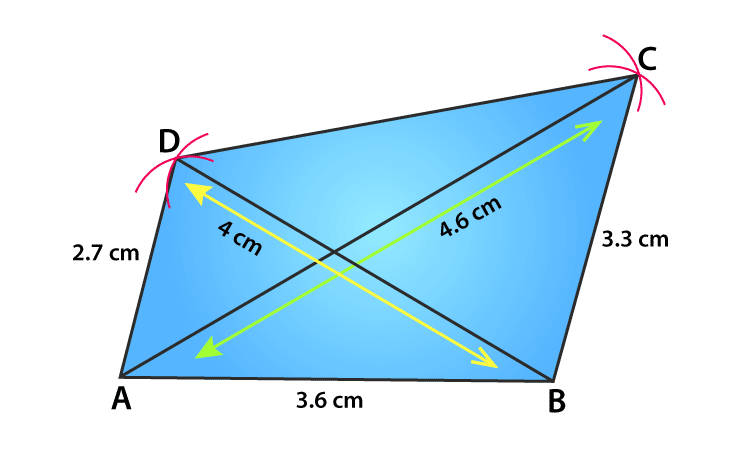5. Construct a quadrilateral PQRS in which QR=7.5cm, PR=PS=6cm, RS=5cm, and QS=10cm. measure the fourth side.

Solution: The given Quadrilateral PQRS can be drawn by following the steps.

Step1: Draw a line QR= 7.5cm

Step2: Draw an arc of 10cm from Q

Step3: From R with 5cm as radius cut the arc drawn in step2 and that point of intersection is S.

Step4: Draw another arc from R with 6cm of radius

Step5: From S take 6cm as radius and cut the arc drawn in step4 and the point of intersection is P. now join PR and PS.

∴ The constructed quadrilateral looks like: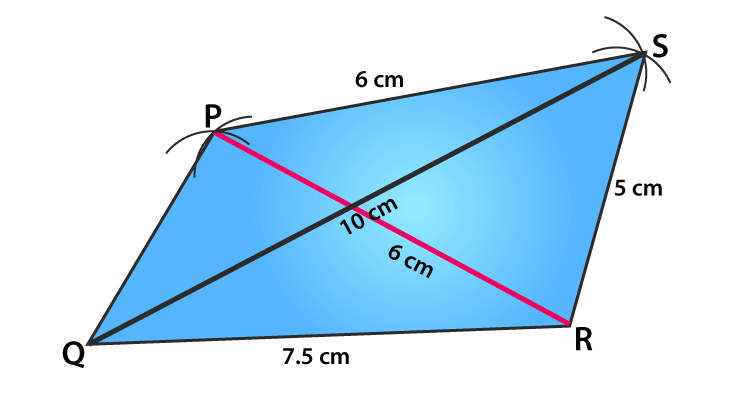6. Construct a quadrilateral ABCD in which AB=3.4cm, CD=3cm, DA=5.7cm, AC=8cm and BD= 4cm.

Solution: The given Quadrilateral ABCD can be drawn by following the steps.

Step1: Draw a line BD= 4cm

Step2: Draw an arc of 3.4cm from B

Step3: from D take radius of 5.7cm and cut the arc drawn in step2 and that point of intersection is A.

Step4: Draw another arc from D with 3cm as radius.

Step5: From A take 8cm and cut the arc drawn in step4 and the point of intersection is C. now join CD and AC.

∴ The constructed quadrilateral looks like: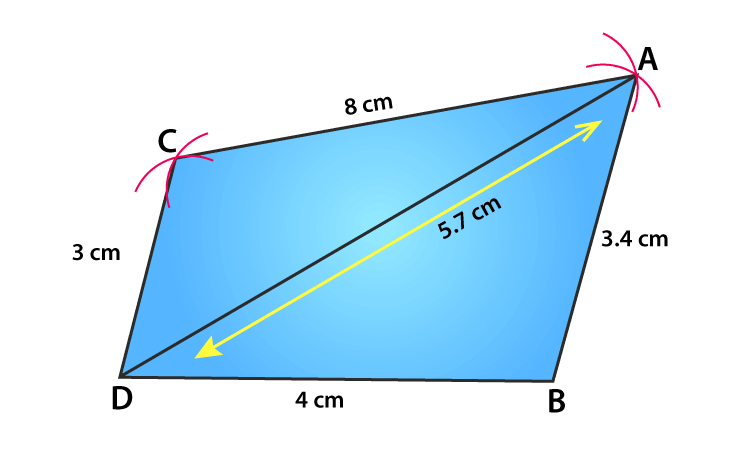## Exercise 17B

1. Construct a parallelogram ABCD in which AB=5.2cm, BC=4.7cm and AC=7.6cm

Solution: The given Parallelogram ABCD can be drawn by following the steps.

Step1: Draw a line AB= 5.2cm

Step2: Draw an arc of 4.7cm from B

Step3: From A take radius of 7.6cm and cut the arc drawn in step2 and that point of intersection is C. join BC and AC.

Step4: Draw another arc from A with 4.7cm as radius.

Step5: From B take 7.6cm and cut the arc drawn in step4 and the point of intersection is D. now join CD and AD.

∴ The constructed parallelogram looks like: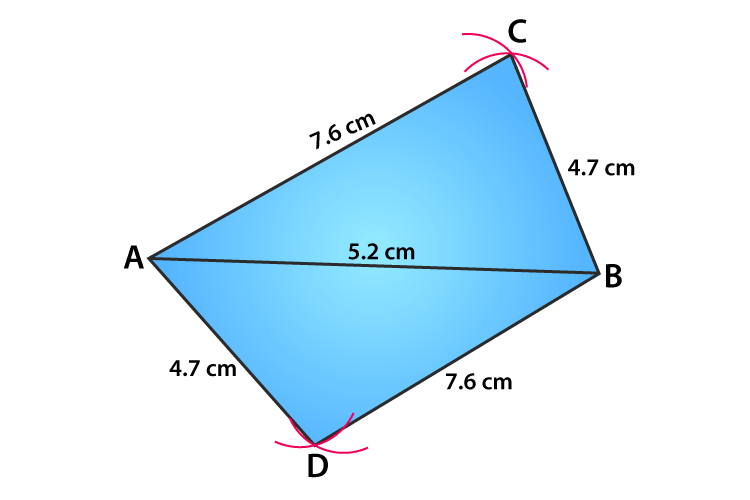2. Construct a parallelogram ABCD in which AB=4.3cm, AD=4cm and BD=6.8cm

Solution: The given Parallelogram ABCD can be drawn by following the steps.

Step1: Draw a line AB= 4.3cm

Step2: Draw an arc of 6.8cm from B

Step3: From A take radius of 4cm and cut the arc drawn in step2 and that point of intersection is D. join AD and BD.

Step4: Draw another arc from B with 4cm as radius.

Step5: From A take 6.8cm and cut the arc drawn in step4 and the point of intersection is C. now join BC and AC.

∴ The constructed parallelogram looks like: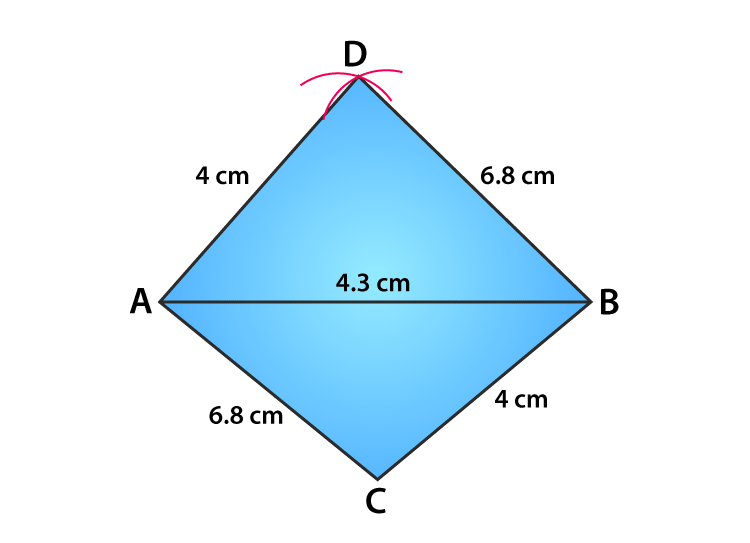3. Construct a parallelogram PQRS in which QR=6cm, PQ=4cm and ∠PQR=60o

Solution: The given Parallelogram ABCD can be drawn by following the steps.

Step1: Draw a line QR= 6cm

Step2: At Q draw ∠RQX= 60o

Step3: Cut PQ= 4cm at the ray QX

Step4: Draw another arc from P with 6cm as radius.

Step5: From R take 4cm and cut the arc drawn in step4 and the point of intersection is S. now join SP and SR.

∴ The constructed parallelogram looks like: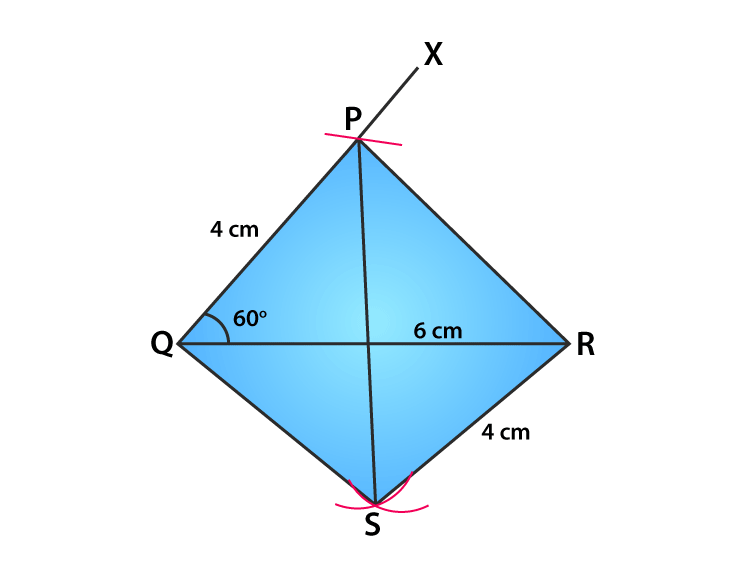4. Construct a parallelogram ABCD in which BC=5cm, ∠BCD=120o and CD=4.8cm.

Solution: The given Parallelogram ABCD can be drawn by following the steps.

Step1: Draw a line BC= 5cm

Step2: At C draw ∠BCX= 120o

Step3: Cut CD= 4.8cm at the ray QX

Step4: Draw another arc from B with 4.8cm as radius.

Step5: From D take 5cm and cut the arc drawn in step4 and the point of intersection is A. now join AB and AD.

∴ The constructed parallelogram looks like: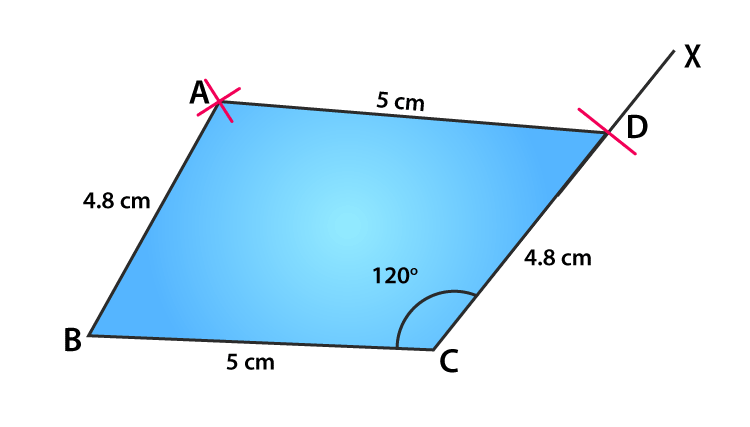5. Construct a parallelogram, one of whose sides is 4.4cm and whose diagonals are 5.6cm and 7cm. measure the other side.

Solution: The given Parallelogram ABCD can be drawn by following the steps.

Step1: Draw a line AB= 4.4cm

Step2: Draw an arc of 2.8cm from B

Step3: From A take radius of 3.5cm and cut the arc drawn in step2 and that point of intersection is O. join OA and OB.

Step4:Extend AO to C where, OC=AO and also extend BO to D where, OD=OB.

Step5: Join AD, BC and CD

∴ The constructed parallelogram looks like: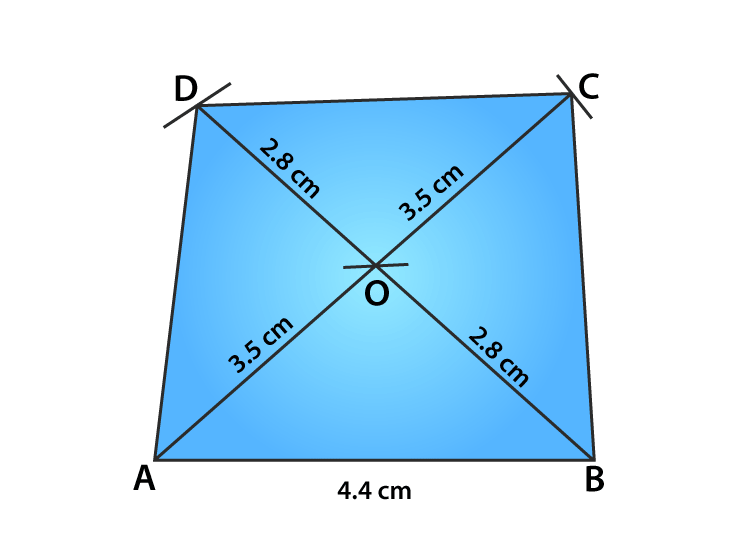6. Construct a parallelogram ABCD in which AB=6.5cm, AC=3.4cm, and the altitude AL from A is 2.5cm. Draw the altitude from C and measure it.

Solution: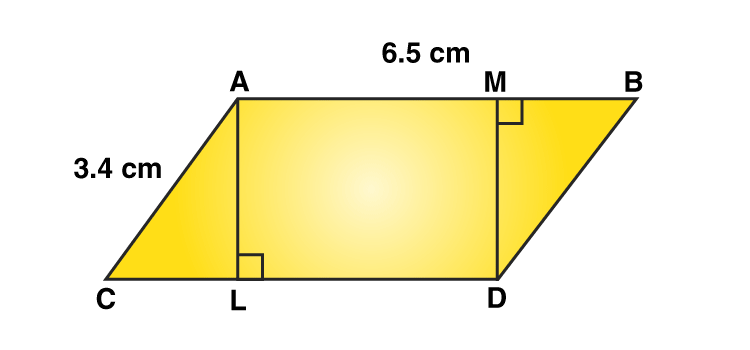We know, AB=6.5cm, AC=3.4cm, AL=2.5cm

From the above figure the construction is not possible. So we have to find the altitude using from D.

Length of the perpendicular from A on DC= AL= 2.5cm (since the perpendicular length is the same on both side).

∴ The length of the perpendicular from D on AB=DM= 2.5cm.

7. Construct a parallelogram ABCD, in which diagonal AC=3.8cm, diagonal BD=4.6cm and the angle between AC and BD is 60o

Solution: The given Parallelogram ABCD can be drawn by following the steps.

Step1: Draw a line AC= 3.8cm

Step2:Bisect AC at O.

Step3: At O draw ∠COX= 60o

Step4: Set the value OB= 2.3cm and OD=2.3cm (divided the value 4.6 into 2.3 and 2.3)

Step5: Now join AB,BC,CD,DA

∴ The constructed parallelogram looks like: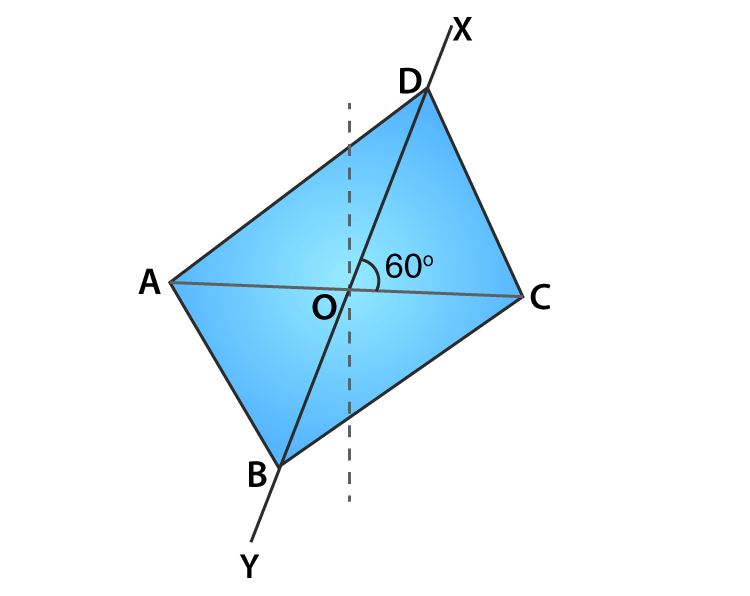8. Construct a rectangle ABCD whose adjacent sides are 11cm and 8.5cm.

Solution: The given rectangle ABCD can be drawn by following the steps.

Step1: Draw a line AB= 11cm

Step2: Draw BE perpendicular to AB

Step3: With 8.5cm from B draw an arc cutting BE at C.

Step4:With 8.5cm from A draw an arc

Step5: From C take 11cm of radius and cut the arc drawn in step4 and the point of intersection is D. now join CD and AD.

∴ The constructed parallelogram looks like: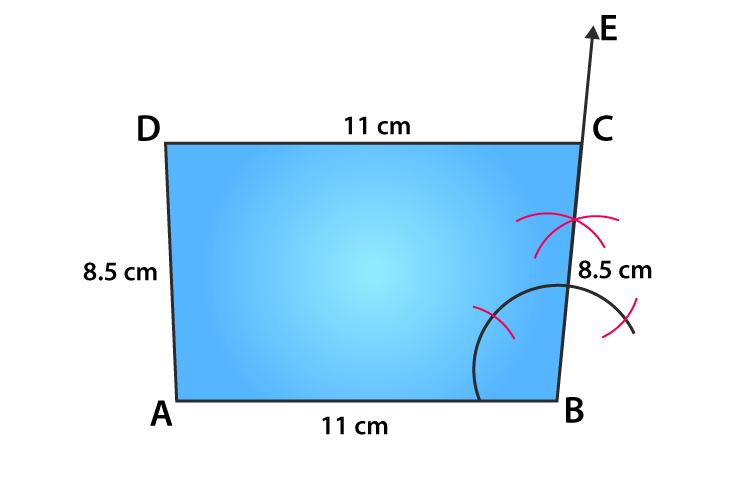## RS Aggarwal Solutions for Class 8 Maths Chapter 17 – Construction of Quadrilaterals

Chapter 17- Construction of Quadrilaterals contains 2 exercises and the RS Aggarwal solutions present on this page provide the solutions for the questions present in each exercise. Now, let us have a look at the concepts discussed in this chapter.

• To construct a Quadrilateral when 4 sides and 1 diagonal are given.
• To construct a Quadrilateral when 3 sides and 2 diagonal are given.
• To construct a Quadrilateral when 3 sides and 2 included angles are given.
• To construct a Quadrilateral when 2 adjacent sides and 3 angles are given.
• To construct a Quadrilateral when 4 sides and 1 angle are given.
• Construction of special types of Quadrilaterals.

Exercise 17A

Exercise 17B

### Chapter Brief of RS Aggarwal Solutions for Class 8 Maths Chapter 17 – Construction of Quadrilaterals

RS Aggarwal Solutions for Class 8 Maths Chapter 17 – Construction of Quadrilaterals, ensures that the students are thorough and familiar with the concepts. Regular revision of important concepts and formulas over time to time is the best way to strengthen your concepts.

As the Chapter is about Construction of Quadrilaterals, we learn how to construct a Quadrilateral by following certain steps provided. In the RS Aggarwal Solutions book, many such exercise problems are given which enhances familiarity with the concepts.